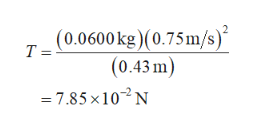A small block with a mass of 0.0600 kg is attached to a cord passing through a hole in a frictionless, horizontal surface (Figure 1). The block is originally revolving at a distance of 0.43 m from the hole with a speed of 0.75 m/s . The cord is then pulled from below, shortening the radius of the circle in which the block revolves to 0.19 m . At this new distance, the speed of the block is 1.70 m/s .What is the tension in the cord in the original situation when the block has speed v0 = 0.75 m/s ?What is the tension in the cord in the final situation when the block has speed v1 = 1.70 m/s ?How much work was done by the person who pulled on the cord?

Question

A small block with a mass of 0.0600 kg is attached to a cord passing through a hole in a frictionless, horizontal surface (Figure 1). The block is originally revolving at a distance of 0.43 m from the hole with a speed of 0.75 m/s . The cord is then pulled from below, shortening the radius of the circle in which the block revolves to 0.19 m . At this new distance, the speed of the block is 1.70 m/s .

What is the tension in the cord in the original situation when the block has speed v0 = 0.75 m/s ?
What is the tension in the cord in the final situation when the block has speed v1 = 1.70 m/s ?
How much work was done by the person who pulled on the cord?
Step 1

The tension in the cord when the object is revolving in a circle of radius r with a speed v is equal to the centripetal force and is expressed as,

Step 2

The tension in the cord if the speed of the block is 0.75m/s can be calculated using equation 1.help_outlineImage Transcriptionclose(0.0600kg) (0.75m/s) (0.43 m) T = 7.85 X102N fullscreen
Step 3

The tension in the cord if the speed of the block is...

Want to see the full answer?

See Solution

Want to see this answer and more?

Our solutions are written by experts, many with advanced degrees, and available 24/7

See Solution
Tagged in

Physics